# Catalan's conjecture

## Statement

This conjecture states that the solution set to Catalan's Diophantine problem:,

forpositive integers not equal to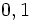andpositive integers greater than one, has precisely one solution: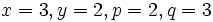.

The conjecture has been proved.

## Relation with other facts/conjectures

Conjecture Statement of conjecture Status
Fermat-Catalan conjecture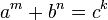has only finitely many solutions forpositive integers and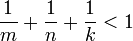open
abc conjecture For every, there exists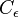such that if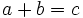, then, the product overprime